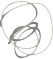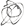Noppanit

02 Sep 2010

# Tutorial : Java Random with scaling

I have been playing around with Random class in Java. Unfortunately, this class doesn’t provide us some methods we need. Such as, limit the range between -50,50 or get random double from 1 – 1000. Something like this we can fine tune. I copied some good practice from this website.

Here are some examples of these operations:

• Suppose you are writing a game program that simulates throwing dice, and so need a random integer in the range 1 to 6. “nextInt” can give you one in the range 0 to 5, and you can translate this to the range you need:

int throw = generator.nextInt(6) + 1;

• In drawing a pattern made up of random lines, you want to pick a random angle between 0 and 360 degrees at which to draw a line. The angle can be any real number. The “nextDouble” message will give you a random real number, but between 0 and 1. You can use scaling to turn this into a real number between 0 and 360:

double angle = generator.nextDouble() * 360.0;

• Suppose the same pattern-drawing program also needs to pick random lengths for the lines, but that the lines should never be shorter than 10 units, nor longer than 50. Line lengths can be any real number between these limits. Thus you need random lengths from a 40-unit range starting at 10. You can use scaling and translation together to generate these numbers from “nextDouble”:

double length = generator.nextDouble() * 40.0 + 10.0;

Til next time,
noppanit at 00:00